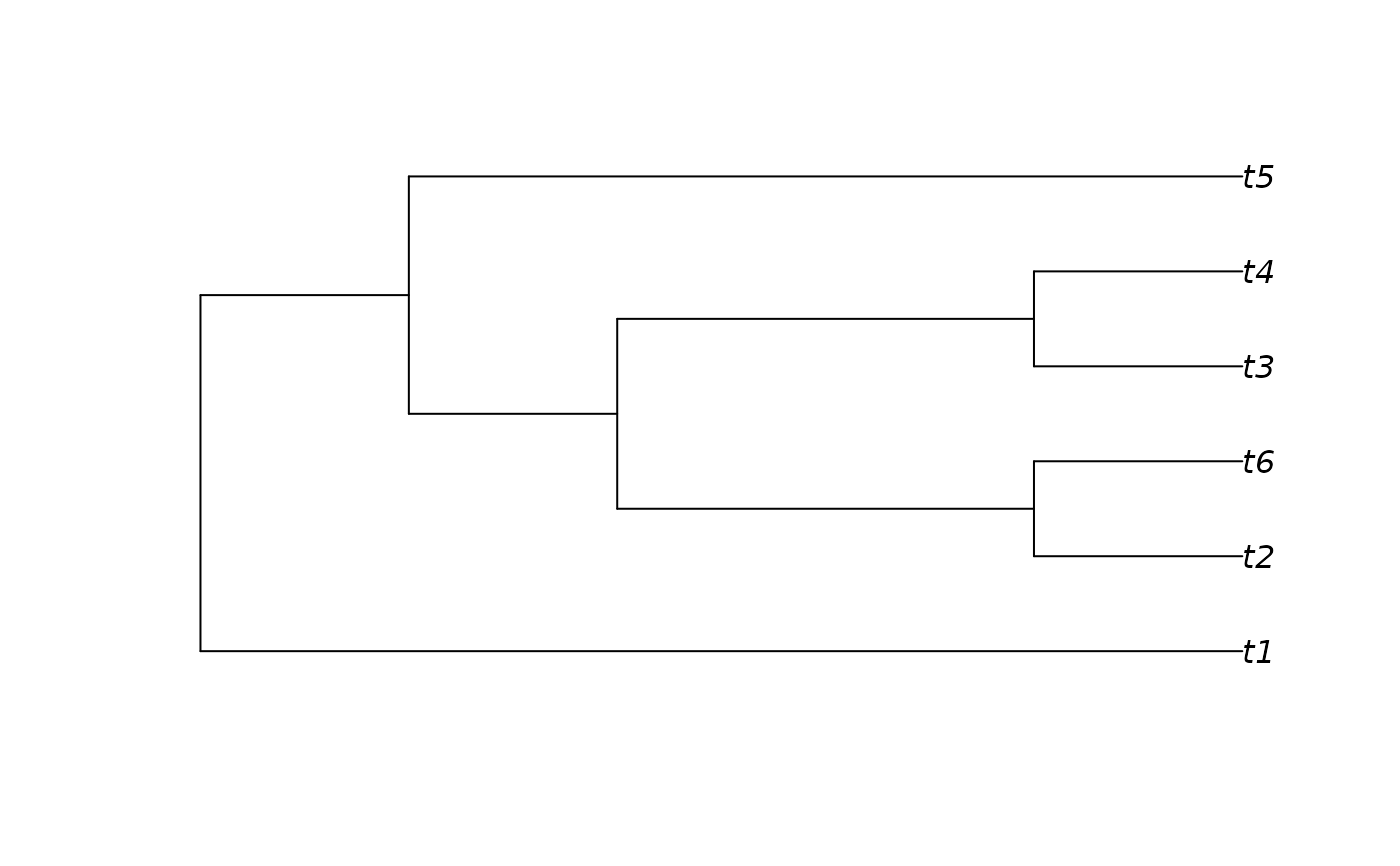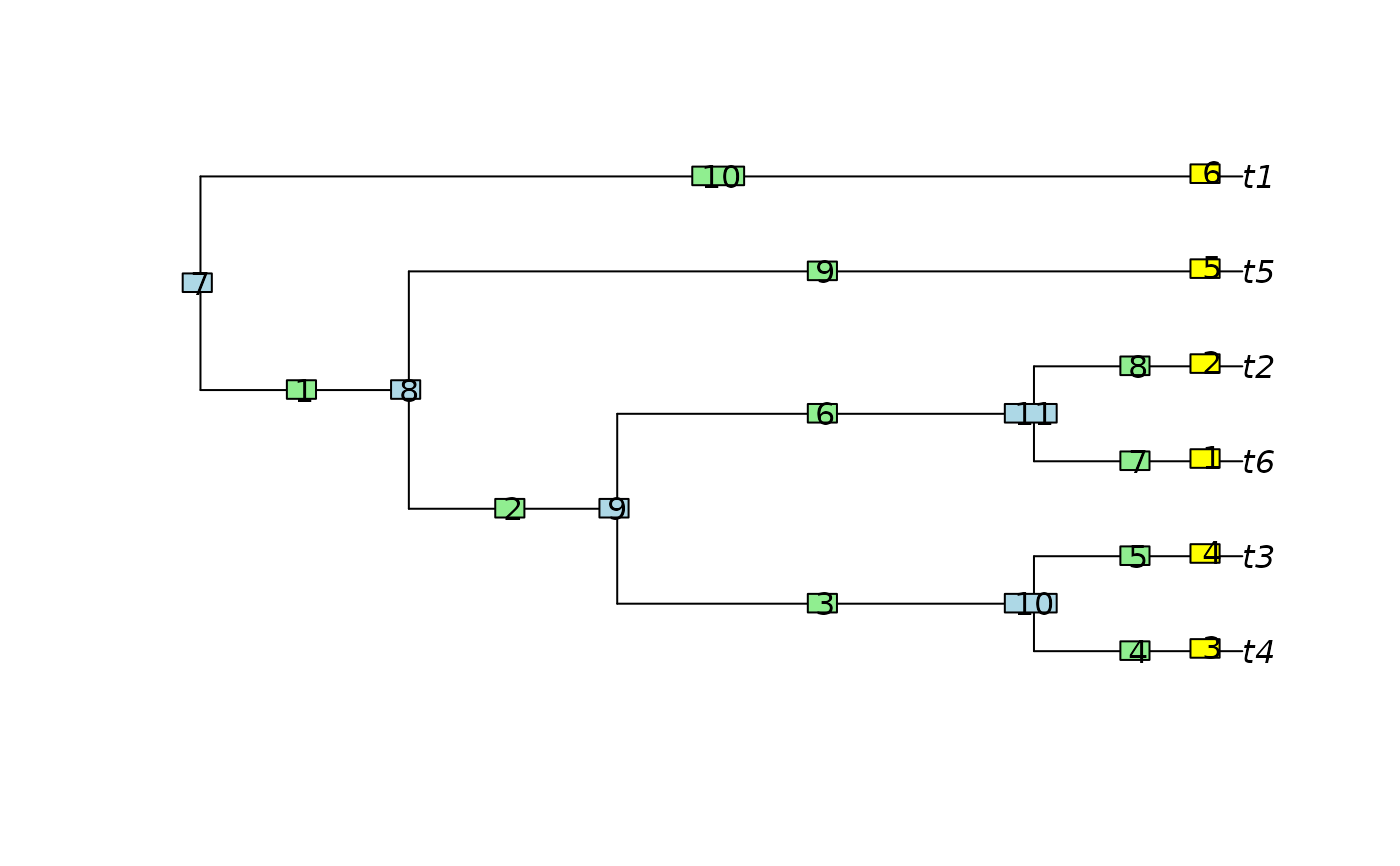SortTree() sorts each node into a consistent order, so that node rotation does not obscure similarities between similar trees.

SortTree(tree, how = "cladesize", order = TipLabels(tree))

# S3 method for phylo
SortTree(tree, how = "cladesize", order = TipLabels(tree))

# S3 method for list
SortTree(tree, how = "cladesize", order = TipLabels(tree[]))

# S3 method for multiPhylo
SortTree(tree, how = "cladesize", order = TipLabels(tree[]))

## Arguments

tree

One or more trees of class phylo, optionally as a list or a multiPhylo object.

how

Character vector specifying sort method: "Cladesize" rotates each node such that the larger clade is first, thus appearing lower when plotted; "TipLabels" rotates nodes such that labels listed sooner in order are listed first, and thus plot lower.

order

Character vector listing tip labels in sequence they should appear on tree. Clades containing a taxon earlier in this list will be listed sooner and thus plot lower on a tree. Taxa not listed in order will be treated as if they were last in the list.

## Value

SortTree() returns tree in the format of tree, with each node in each tree sorted

## Details

At each node, clades will be listed in tree[["edge"]] in decreasing size order.

Clades that contain the same number of leaves are sorted in decreasing order of minimum leaf number, so (2, 3) will occur before (1, 4).

As trees are plotted from "bottom up", the largest clades will "sink" to the bottom of a plotted tree.

Preorder() also rearranges trees into a consistent shape, based on the index of leaves.

sort.multiPhylo() sorts a list of trees stored as a multiPhylo object.

Other tree manipulation: AddTip(), CollapseNode(), ConsensusWithout(), DropTip(), EnforceOutgroup(), ImposeConstraint(), KeptPaths(), KeptVerts(), LeafLabelInterchange(), MakeTreeBinary(), RenumberTips(), RenumberTree(), Renumber(), RootTree(), Subtree(), TipTimedTree(), TrivialTree

## Examples

messyTree <- as.phylo(10, 6)
plot(messyTree)sorted <- SortTree(messyTree)
plot(sorted)
ape::nodelabels()
ape::edgelabels()